# Kulraj Suri

Software Engineer / Quant Dev

# A Simple Julia Optimisation Problem

1. What is Optimisation?

• The concept of producing the best possible result with the available resources
• Optimisation is the process of minimising or maximising something
• It is an iterative process
• E.g. looking for the maximum point on the mountain: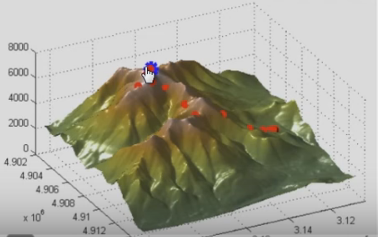2. The Physical Problem• One boy bets the other that he can locate the top of the hill blindfolded
• The other boy agrees but asks the first boy to also stay inside the fences
• Translating this situation into an optimisation formulation, we see that the objective is to find the highest point on the hill
• Therefore the objective function is the height achieved by the first boy with respect to his original position
• The variables of this objective function could be longitude and latitude (x and y), defining the position of the boy
• The constraints are that the boy has to stay inside the fences
• It is possible to define this problem mathematically

3. The Engineering Problem

• Our objective is to find the highest point on the hill, so our objective function would be a curve (or 3-D surface) defining the hill itself
• We can define a function for the hill like so:• Mathematically the hill is a graph in 3 dimensions; x (= X1), y (= X2) and z (=Y)
• It could look something like this:• Next, we need to define our constraints which in this case would be two functions representing the two fences on the hill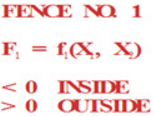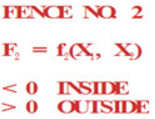4. Formulating the Problem

• Maximize the objective function:• Subject to the constraints:5. The Optimisation Process

• The optimisation process can be broken down into:
• Find a search direction that will improve the objective (find the highest point on the hill) while staying inside the fences
• Search in this direction until no more improvement can be made by going in this direction
• Repeat the process, until no search can be found that improves the objective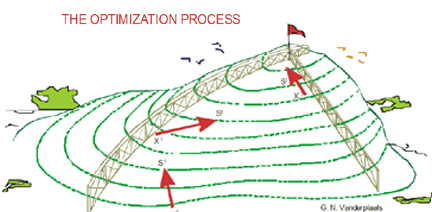• There are many different algorithms available that search for the ‘optimum point’, each with a different method of getting there.
• Some are better suited to the problem than others as they require less iterations to find the optimum point; reducing time, memory & cpu usage
• This will depend on the problem itself:6. Optimisation Workflow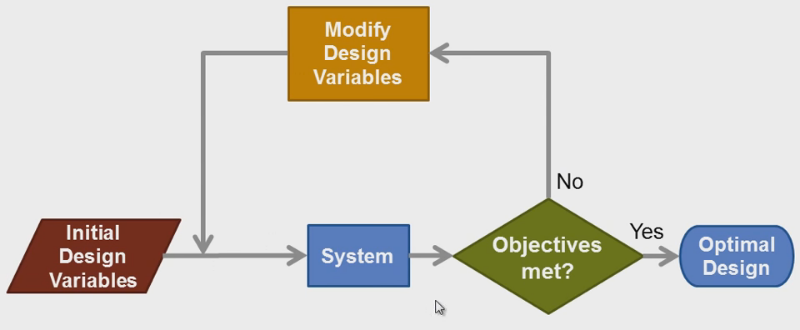7. Benefits of Optimisation

• Finding better (optimal) designs
• Faster design evaluations
• Useful for trade-off analysis
• Non-intuitive designs may be found

8. Other Examples

• If we wish to design the internal combustion engine, the objective may be to maximise the combustion efficiency
• The engine may be required to provide a specific power output with an upper limit on the amount of harmful pollutants emitted into the atmosphere
• These parameters will serve as constraints for optimisation
• The variables that are allowed to be changed during optimisation may be the compression ratio, air-fuel mixture ratio, bore and stroke, etc
• You could also optimise:
• The route of a delivery truck
• A financial portfolio
• Protein models

9. Final Project: A Simple Optimisation Problem

The Problem

• We need to enclose a field with a fence
• We have 500 metres of fencing material and a building is on one side of the field and so won’t need any fencing
• Determine the dimensions of the field that will enclose the largest area

Sketching the Problem

• In this problem we have two functions:
• Objective function
• Constraint function
• Sketching the situation will help us to arrive at these equations: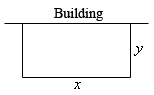• In this problem we want to maximise the area of a field and we know that it will use a maximum of 500 metres of fencing material
• The area of the field will be the function we are trying to optimise and the amount of fencing is the constraint:• Now we are ready to model and solve our problem in Julia

Setting Up Our Julia Environment

• Before we jump into the code, we need to install the relevant Julia optimisation packages
• Firstly we shall install JuMP; an algebraic modelling language for linear, quadratic and nonlinear constrained optimisation problems embedded in Julia
• Open the Julia application and in the command prompt enter:• Next we shall install Ipopt; a nonlinear optimisation solver using the interior point algorithm to iterate to the optimum pointCreate the Julia File

• Create a .jl julia file in a text editor – I have saved mine as “ example.jl “• First, it helps to write the problem in mathematical form as comments in the code
• This will help when coding the problem and also help others who may view your code to understand what you are trying to achieve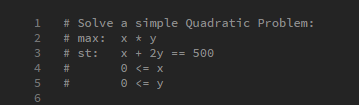• From this it is clear we are trying to solve an optimisation problem

Import Packages

• Next we need to include the two packages (JuMP and Ipopt) in our example.jl file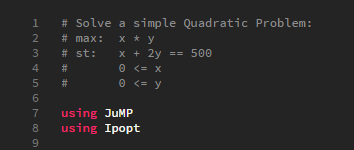• Now all of the functions from the aforementioned packages are available to us when we run our example.jl script

Create a Model

• Next we need to define our Model, which we will name “ m “• Models are Julia objects and all variables and constraints are associated with a Model object
• Also, in the argument we state the solver we are going to use for this particular problem (there are many other solvers available – see here)

Define Variables

• Now we need to define the variables of our problem and their bounds
• The first argument will always be the Model to associate this variable with• As we can see from the comments (lines 1-5), clearly the variables are x and y and both have a lower bound of 0 (line 4-5)

Objective Function

• With JuMP it is very easy to define the objective function• The first argument will always be the Model to associate this function with
• The second argument is whether we are trying to find the minimum (Min) or maximum (Max) optimal solution
• Finally the third argument is the objective function itself

Constraints

• As with the objective function, JuMP makes it very simple to define constraints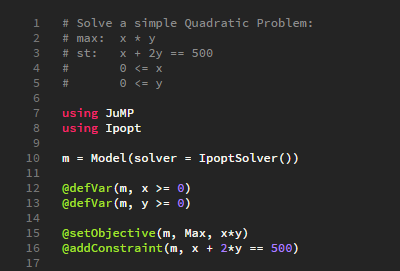• The first argument will always be the Model to associate this function with
• The second argument is the constraint
• It is worth noting that we can define multiple constraints in this way

Print Model

• When we run our ‘example.jl’ Julia script, it would be useful if we could see what our model looks like before the optimising (solving) process begins• The print function is defined for models and allows us to see our model looks like in a human readable format
• When we run our example.jl file the model will look like this:Solve

• Models are solved with the solve() function
• This function will not raise an error if your model is infeasible – instead it will return a flag
• In this case, the model is feasible so the value of status will be :OptimalPrint Results

• Finally we can access the results of our optimisation
• Getting the values for the objective function and x & y variables is simple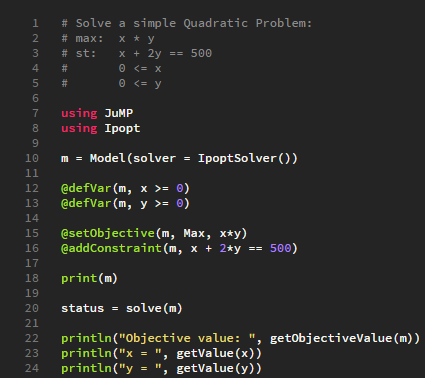Solution

• Now we are ready to save and run our ‘example.jl’ script
• Open a Julia command prompt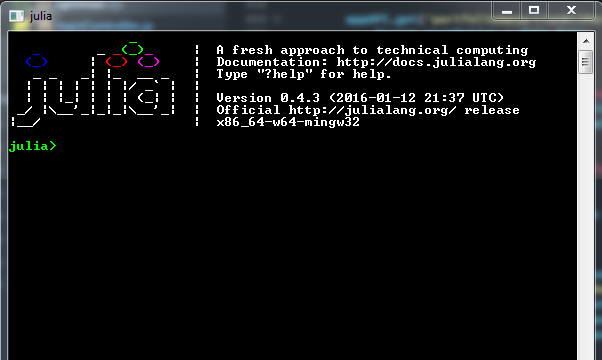• Locate the directory where ‘example.jl’ is saved using cd()
• Remember to use “ \\ “ when entering the directory• Now to run the ‘example.jl’ script we use include()• Finally we have our results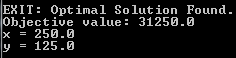Results

• The original question was to determine the maximum possible area of the field given we have 500 metres of fencing material and there is a building on one side of the field so won’t need any fencing• We can see from running our ‘example.jl’ Julia script that the maximum area of the field is 31,350 m²
• We can clearly see the values for x (250 m) and y (125 m) satisfy the constraint x+2y = 500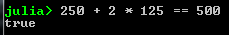## 1 Comment

1.messenger

Thanks fօr the marvelous posting! I genuinely enjoyed reading it,
you can be a great author. I will be sure tо bookmark your blog and will often come back from now on. I want to encourage you to
ultimately continuе your great writing, have a nice morning!